### Become an OU studentGeometry

Start this free course now. Just create an account and sign in. Enrol and complete the course for a free statement of participation or digital badge if available.

# 2.8 The angles of a triangle

The sum of the angles of any triangle is 180°. This property can be demonstrated in several ways. One way is to draw a triangle on a piece of paper, mark each angle with a different symbol, and then cut out the angles and arrange them side by side, touching one another as illustrated.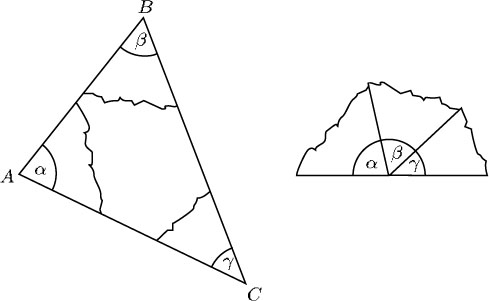You can see why it is that the angles fit together in this way by looking at the triangle below. An extra line has been added parallel to the base. The angle of the triangle,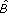, is equal to the angle β at the top (they are alternate angles), and similarly the angle of the triangle,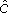, is equal to the angle γ at the top (they are also alternate angles). The three angles at the top (β, γ and the angle of the triangle,) form a straight line of total angle 180°, and so the angles of the triangle must also add up to 180°.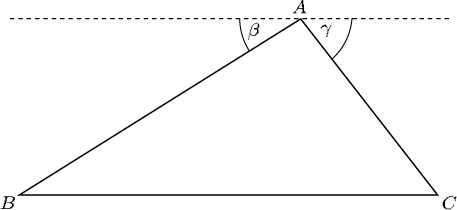The sum of the angles of a triangle is 180°.

The fact that the angles of a triangle add up to 180° is another angle property that enables you to find unknown angles.

## Example 7

Find α, β and θ in the diagram below.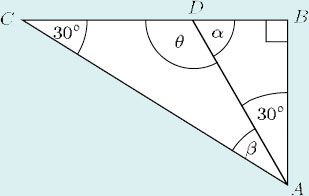First, look at the angles of ΔABD: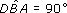and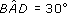Then, by the angle sum property of triangles,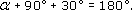So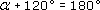and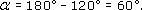As CDB is a straight line and α = 60°, it follows that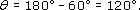Now consider the angles of ΔADC: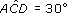and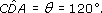Therefore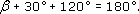So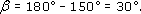(Check for yourself that the angles of ΔABC also add up to 180°.)

In a right-angled triangle, since one angle is a right angle (90°), the other two angles must add up to 90°. Thus, in the example below, α + β = 90°.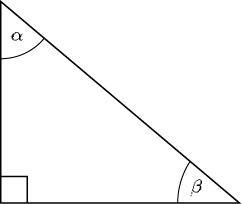In an equilateral triangle, all the angles are the same size. So each angle of an equilateral triangle must be 180° ÷ 3 = 60°.

In an isosceles triangle, two sides are of equal length and the angles opposite those sides are equal. Therefore, α = β in the triangle below.

Such angles are often called base angles.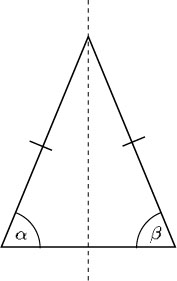This means that there are only two different sizes of angle in an isosceles triangle: if the size of one angle is known, the sizes of the other two angles can easily be found. The next example shows how this is done.

## Example 8

Find the unknown angles in these isosceles triangles, which represent parts of the roof supports of a house.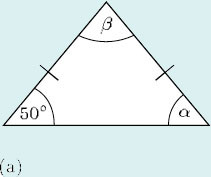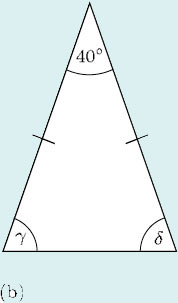• (a) As α and 50° are the base angles, α = 50°. By the angle sum property of triangles,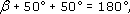therefore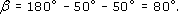• (b) As γ and δ are the base angles, γ = δ. In this triangle,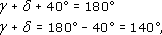therefore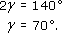The various angle properties can also be used to find the sum of the angles of a quadrilateral.

## Example 9

The diagram below represents the four stages of a walk drawn on an Ordnance Survey map.

The figure ABCD is a quadrilateral. Find θ and φ, and thus the sum of all the angles of the quadrilateral.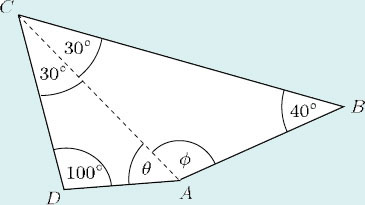From ΔABC,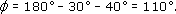From ΔACD,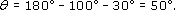Then the sum of all the angles of the quadrilateral is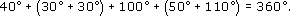In fact, you can find the sum of the four angles of a quadrilateral without calculating each angle as in Example 9. Look again at the quadrilateral: the dotted line splits it into two triangles, and the angles of these triangles together make up the angles of the quadrilateral. Each triangle has an angle sum of 180°, so the angle sum of the quadrilateral is 2 × 180° = 360°. This is true for any quadrilateral.

The sum of the angles of a quadrilateral is 360°.

Similarly, other polygons (that is, other shapes with straight sides) can be divided into triangles to find the sum of their angles.